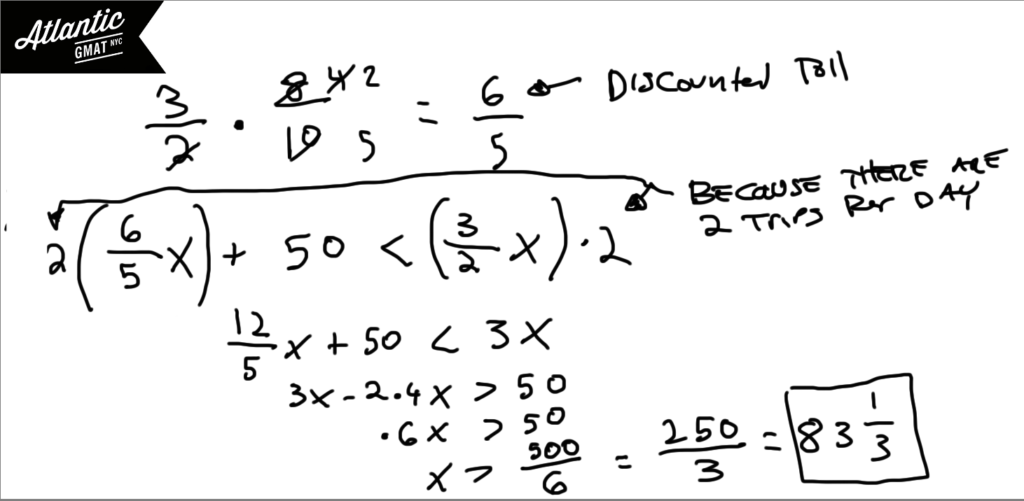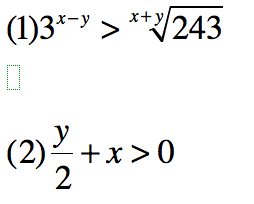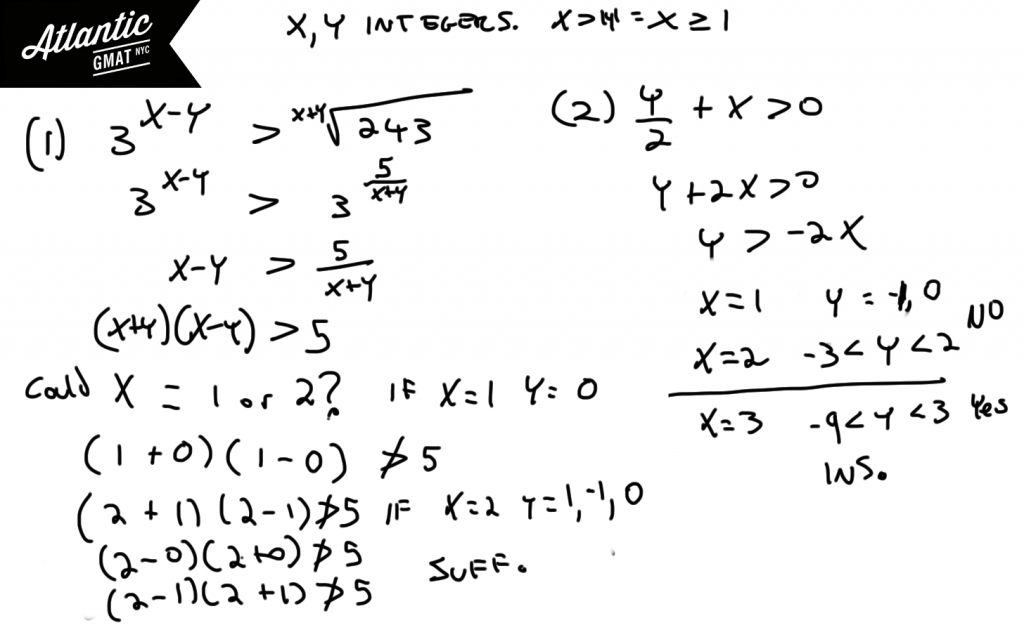Private GMAT Tutoring in NYC & Online

The rate of a certain chemical reaction is directly proportional to the square of the concentration of chemical A present and inversely proportional to the concentration of chemical B present. If the concentration of chemical B is increased by 100 percent, which of the following is closest to the percent change in the concentration of chemical A required to keep the reaction rate unchanged?

(A) 100% decrease
(B) 50% decrease
(C) 40% decrease
(D) 40% increase
(E) 50% increase

Let's define the question: which of the following is closest to the percent change in the concentration of chemical A required to keep the reaction rate unchanged? So B is getting doubled. What do we need to do to A to stay in the same place. The chemical reaction increases by the square of A and decreases by B. So we need to take the square root of whatever the B increase is (because that number will end up getting squared). Again, B is doubled. So that means it's multiplied by 2. So we need A to increase by √2 in order to stay even because that √2 will be squared, produce 2 which will cancel out the decrease caused by B. √2 is approximately 1.4 so a 40% increase. Choose D.

If xy + z = x(y + z), which of the following must be true?

(A) x = 0 and y = 0
(B) x = 1 and y = 1
(C) y = 1 and z = 0
(D) x = 1 or y = 0
(E) x = 1 or z = 0

# GMAT Question of the Day - Problem Solving - Word Problem/Algebra

On his daily commute Bill always crosses a certain toll bridge exactly two times. Bill can buy a discount pass for \$50 which decreases the cost of the toll by 20%. If the toll costs \$1.50 per crossing, after how many days of commuting will buying the discount pass result in a financial benefit for Bill?

A. 81

B. 82

C. 83

D. 84

E. 85

## GMAT Question of the Day Solution

This question of the day is a GMAT classic. It is likely that you will see this style question on your exam. There are few things to think about with GMAT word problems:

1. Read carefully. Work on processing and understanding the information. Don't worry about solving while you are reading.

2. Define the question. Ask: What am I looking for?

3. As you are working towards the solution look for ways to simplify your arithmetic by factoring and reducing.# GMAT Question of the Day - Data Sufficiency - Absolute Value/Algebra

x and y are integers. If x > |y| is x > 2?## GMAT Question of the Day Solution

GMAT absolute value questions generally focus on the polarity of the variables. Think of what happens when things are positive and when things are negative. In this case we know that x will be positive because of the given information. Now we have to decide whether x will be greater than 2. The first statement proves that x will in fact be greater than two. The second leaves open many possibilities. If you struggled with the exponents/roots in the first statement it's time to review your exponent rules.# GMAT Question of the Day - Data Sufficiency - Percent Change

If the total revenue at Company K and Company M decreased by d% from 2001 to 2003 what is the value of d?

(1) In 2001 Company K earned 2,500,000 more revenue than Company M earned.

(2) In 2003 Company K earned 2,225,000 more revenue than Company M earned.

# GMAT Question of the Day Solution

In this question you will end up with two equations and three variables. Unless there is some special "perfect fit" happening you will be unable to solve for d. There happens to be a perfect fit if you substitute in for k-m. Once you do that you'll just have one variable and one equation.

How to see the perfect fit? In GMAT tutoring I'm always suggesting that students slow down and map out the question. In this case, avoid doing the work in your head. Try to write out the equations. Simplify the equation if you can. Factor. Reduce. The equations may seem intimidating but at the end of the day these equations are pretty standard. If it is challenging to write out these equations then you should consider working a bit more on your algebra. The GMAT is a nuanced critical thinking exam but your fundamentals are still extremely important.

# CONTACT

Atlantic GMAT

405 East 51st St.

NY, NY 10022

(347) 669-3545

info@AtlanticGMAT.com